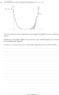# Area between 2 curves

• squenshl
squenshl
Member warned to type the problem statement, not just post an image with type that is too small to read

See attached.

## The Attempt at a Solution

Ok so the first thing you want to do is find the equation of the tangent line which is done in the usual way to get ##y=8x-7##. This cuts the ##x## axis at ##x=\frac{7}{8}## so we know the upper limit of the integral to calculate.
Is ##P## a turning point if not how do I find it?

#### Attachments

•D8BFD390-A952-4AB6-B66E-BF7C83845A30.jpeg
14.2 KB · Views: 258
Last edited by a moderator:

Homework Helper
See attached.
Can't read the very small print. write out a problem statement, sort out what you are supposed to do !

Gold Member
2022 Award
I"m having the same problem. When you ask someone to do you a favor, like answer a question, it's not a good idea to make it really hard for them to help you.

squenshl
I seem to read it fine once I open the attachment.
It is asking given we have ##f(x) = (2x-1)^4##. The curve meets the ##x##-axis at a point ##P## and the line on the graph is a tangent to the curve at the point ##Q(1,1)##.
Find the area of the region bounded by the curve, the ##x##-axis, and the tangent to the curve at ##Q##.

Homework Helper
Dearly Missed
I seem to read it fine once I open the attachment.
It is asking given we have ##f(x) = (2x-1)^4##. The curve meets the ##x##-axis at a point ##P## and the line on the graph is a tangent to the curve at the point ##Q(1,1)##.
Find the area of the region bounded by the curve, the ##x##-axis, and the tangent to the curve at ##Q##.

I cannot read it once I open the attachment.

Anyway, the PF standard is that the person wanting help takes the trouble to type out the problem and the work done so far, reserving attachments for things like diagrams and perhaps tables, etc. Read the Guidelines for more on this issue.

squenshl
Ok I found the equation of the tangent curve in the standard way to get ##y=8x-7## which cuts the x-axis at ##x=\frac{7}{8}##.
To find P I found the x coordinate Of the turning point which is ##x=0.5## so now we have our bounds of our integral so we just calculate ##\int_{0.5}^{7/8} (2x-1)^4-8x+7 \; dx##.
I get ##0.5862##.

Homework Helper
My problem is that I need to set the magnification for Chrome to such a high value that all other screens become useless.
And all that because you don't want to type out the problem statement, which is quite straightforward an clear.
Good thing you found the 0.5 by yourself, as well as the 7/8.
But in the figure I clearly see there is a part of the integral between 7/8 and 1
And I also see the integral between 0.5 and 7/8 is not how you describe it...All this would have come out if you re-typed the problem statement and formulated what to do to find the answer in your own words, which is why using the template is so useful that it is compulsory in PF

Homework Helper
Member warned to type the problem statement, not just post an image with type that is too small to read

See attached.

## The Attempt at a Solution

Ok so the first thing you want to do is find the equation of the tangent line which is done in the usual way to get ##y=8x-7##. This cuts the ##x## axis at ##x=\frac{7}{8}## so we know the upper limit of the integral to calculate.
Is ##P## a turning point if not how do I find it?
P is the point where the curve meets the x axis. Can you find it?

squenshl
P is the point where the curve meets the x axis. Can you find it?
Yes it’s ##x=0.5##.

Homework Helper
Good. That is the lower bound of the integral. What is the upper bound ?

squenshl
Good. That is the lower bound of the integral. What is the upper bound ?
I’m thinking it’s where the tangent function cuts the ##x##-axis which is when ##8x-7=0##, or ##x=\frac{7}{8}##.

squenshl
Oops no it isn’t that will be ##x=1##.

squenshl
So that means I solve ##\int_{0.5}^{1} (2x-1)^4 -8x + 7 \; dx## in which case I get ##0.6##?

Homework Helper
Don't jump to conclusions -- they aren't interesting anyway. Do you see a difference between the regions 0.5 -- 7/8 and 7/8 -- 1 ?

Homework Helper
So that means I solve ##\int_{0.5}^{1} (2x-1)^4 -8x + 7 \; dx## in which case I get ##0.6##?
As a kind of check:
Cut a piece of paper of 1 in x and 0.6 in y and compare its area with the shaded areasquenshl
As a kind of check:
Cut a piece of paper of 1 in x and 0.6 in y and compare its area with the shaded areaHold up.
I can just calculate ##\int_{0.5}^{1} (2x-1)^4 \; dx=0.1##.
Then calculate ##\int_{7/8}^{1} 8x-7 \; dx=0.0625##.
Now take the difference to get ##\frac{3}{80}=0.0375##.